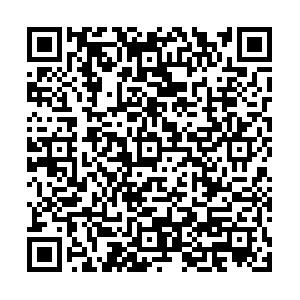## 留言板引用本文: 赵宏泽, 魏光辉, 杜雪, 等. 雷达装备二阶互调伪信号干扰效应预测模型[J]. 强激光与粒子束, 2023, 35: 083001.Zhao Hongze, Wei Guanghui, Du Xue, et al. Prediction model of second-order intermodulation pseudo-signal interference effect for radar equipment[J]. High Power Laser and Particle Beams, 2023, 35: 083001. doi: 10.11884/HPLPB202335.230089
 Citation: Zhao Hongze, Wei Guanghui, Du Xue, et al. Prediction model of second-order intermodulation pseudo-signal interference effect for radar equipment[J]. High Power Laser and Particle Beams, 2023, 35: 083001.• 中图分类号: O441

## Prediction model of second-order intermodulation pseudo-signal interference effect for radar equipment

• 摘要: 为掌握雷达装备的抗电磁干扰性能，需要对其进行全面的电磁环境效应测试与评估，为雷达应用及电磁防护加固提供技术支撑。从二阶互调电磁辐射伪信号干扰效应机理出发，引入效应指数的概念将复杂电磁环境适应性的多变量问题用单一变量表征，实现了雷达装备复杂电磁环境伪信号干扰效应的定量表征，建立了二阶互调伪信号干扰效应评估模型。以某型扫频连续波测距雷达为研究对象，针对受试雷达在试验中出现的二阶互调伪信号干扰现象，给出了模型参数的确定方法，提出雷达装备二阶互调伪信号干扰效应预测评估方法。实验验证结果表明：无论是改变伪信号敏感电平，还是在大辐射频偏、大互调频差范围内改变双频电磁辐射组合，采用普适性二阶互调伪信号干扰效应模型对受试雷达进行二阶互调伪信号干扰效应评估，评估误差均在2.5 dB以内，依据效应预测方法能够客观评估雷达装备二阶互调伪信号干扰。
• 图  1  受试雷达单频电磁辐射临界阻塞干扰场强

Figure  1.  Critical blocking interference field strength of single frequency electromagnetic radiation of the tested radar

图  2  双频电磁辐射作用下雷达探测目标成像

图  3  二阶互调伪信号随干扰场强的变化曲线

Figure  3.  Variation curve of second-order intermodulation pseudo-signal with interference field strength

图  4  干扰场强固定时的二阶互调伪信号电平随辐射频差的变化

Figure  4.  Change of second-order intermodulation pseudo-signal level with radiation frequency difference when the interference field strength is fixed

图  5  二阶互调伪信号干扰因子随辐射频偏的变化

Figure  5.  Change of second-order intermodulation pseudo-signal interference factor with radiation frequency offset

图  6  低频伪信号电平相对值随二阶互调频差的变化

Figure  6.  Curve of the relative value of the low-frequency pseudo-signal level with the second-order cross-frequency modulation difference

表  1  单频电磁辐射临界阻塞干扰场强拟合值

Table  1.   Fitting values of single frequency electromagnetic radiation critical blocking interference field strength

 Δfi/MHz Ei0/(V·m−1) Δfi/MHz Ei0/(V·m−1) Δfi/MHz Ei0/(V·m−1) Δfi/MHz Ei0/(V·m−1) Δfi/MHz Ei0/(V·m−1) −320 90.2 −180 2.1 −40 0.5 100 0.6 240 79.4 −300 88.1 −160 0.9 −20 0.5 120 0.7 260 85.1 −280 76.7 −140 0.5 0 0.5 140 0.8 280 87.1 −260 50.7 −120 0.5 20 0.5 160 1.1 300 89.1 −240 24.3 −100 0.5 40 0.5 180 4.0 320 87.1 −220 11.4 −80 0.5 60 0.6 200 18.2 − − −200 4.9 −60 0.5 80 0.6 220 60.9 − −

表  2  二阶互调伪信号干扰因子$\;{ \beta _{\text{F}}}(\Delta {f_i})$的测试结果

Table  2.   Test results of second-order intermodulation pseudo-signal interference factor $\;{ \beta _{\text{F}}}(\Delta {f_i})$Δfi/MHz $\;{ \beta _{\text{F}}}(\Delta {f_i})$/dB Δfi/MHz $\;{ \beta _{\text{F}}}(\Delta {f_i})$/dB Δfi/MHz $\;{ \beta _{\text{F}}}(\Delta {f_i})$/dB Δfi/MHz $\;{ \beta _{\text{F}}}(\Delta {f_i})$/dB −340 10.3 −160 11.4 20 12.8 200 10.8 −320 10.5 −140 11.9 40 13.0 220 10.4 −300 10.5 −120 12.4 60 13.2 240 10.2 −280 10.4 −100 12.7 80 13.3 260 10.2 −260 10.3 −80 12.8 100 13.3 280 10.2 −240 10.2 −60 12.8 120 13.0 300 10.3 −220 10.2 −40 12.7 140 12.5 320 10.2 −200 10.4 −20 12.6 160 11.9 340 10.1 −180 10.8 0 12.6 180 11.3 360 9.9

表  3  低频伪信号电平相对值Xr(∆f)的测试结果

Table  3.   Test results of low frequency pseudo signal level relative value Xr(∆f)

 |Δf|/MHz Xr(∆f)/dB |Δf|/MHz Xr(∆f)/dB |Δf|/MHz Xr(∆f)/dB |Δf|/MHz Xr(∆f)/dB |Δf|/MHz Xr(∆f)/dB 0.1 0.4 0.7 0.0 1.3 2.4 1.9 6.3 2.5 11.5 0.2 0.5 0.8 0.0 1.4 3.0 2 7.1 2.6 12.9 0.3 0.4 0.9 0.2 1.5 3.7 2.1 7.9 2.7 14.7 0.4 0.4 1 0.5 1.6 4.3 2.2 8.7 2.8 16.9 0.5 0.2 1.1 1.0 1.7 4.9 2.3 9.5 2.9 19.6 0.6 0.1 1.2 1.7 1.8 5.6 2.4 10.4 3 22.7

表  4  不同敏感电平下二阶互调伪信号干扰效应评估

Table  4.   Interference effect evaluation of second-order intermodulation pseudo-signal under different sensitive levels

 criterion/dBmV Δf1/MHz Δf2/MHz βF(Δf1)= βF(Δf2) Xr(0.6M) $(\dfrac{{E}_{1}}{{E}_{10}}\text{.}\dfrac{{E}_{2}}{{E}_{20}})\text{/dB}$ RFS2/dB 6 −0.6 0 12.6 0.1 −30.5 0.6 −0.3 0.3 12.6 0.1 −32.1 −1.0 0 0.6 12.6 0.1 −30.2 0.9 18 −0.6 0 12.6 0.1 −18.6 0.5 −0.3 0.3 12.6 0.1 −19.1 0 0 0.6 12.6 0.1 −18.9 0.2

表  5  大辐射频偏范围二阶互调伪信号干扰效应评估

Table  5.   Interference effect evaluation of second-order intermodulation pseudo-signal in large radiation frequency offset range

 Δfi/MHz βF(Δf2) $(\dfrac{{E}_{1}}{{E}_{10}}\text{.}\dfrac{{E}_{2}}{{E}_{20}})\text{/dB}$ RFS2/dB −300 10.5 −19.1 −1.1 −200 10.4 −18.0 −0.2 −100 12.7 −22.4 0 0 12.6 −22.0 0.2 100 13.3 −23.5 0.1 200 10.8 −19.7 −1.1 300 10.3 −15.7 1.9

表  6  大互调频差范围二阶互调伪信号干扰效应评估

Table  6.   Interference effect evaluation of second-order intermodulation pseudo-signal with large intermodulation frequency difference range

 |Δf|/MHz βF(Δf2) Xr(∆f)/dB $(\dfrac{ {E}_{1} }{ {E}_{10} }\text{.}\dfrac{ {E}_{2} }{ {E}_{20} })\text{/dB}$ RFS2/dB 0.6 12.6 0.1 −21.5 0.6 1.2 12.6 1.7 −19.6 0.9 1.8 12.6 5.6 −17.1 −0.5 2.4 12.6 10.4 −13.5 −1.7 3.0 12.6 22.7 3.0 2.5
•点击查看大图
##### 计量
• 文章访问数:  177
• HTML全文浏览量:  78
• PDF下载量:  31
• 被引次数: 0
##### 出版历程
• 收稿日期:  2023-04-05
• 修回日期:  2023-05-26
• 录用日期:  2023-05-15
• 网络出版日期:  2023-06-01
• 刊出日期:  2023-08-15

### 目录/下载:  全尺寸图片 幻灯片
• 分享
• 用微信扫码二维码

分享至好友和朋友圈